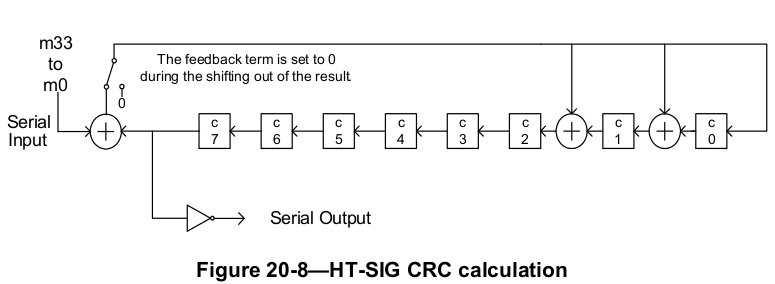The HT-SIG field of 802.11n PLCP preamble contains a 8-bit CRC for the receiver to validate the sanity of the header. Here is how to calculate it.HT-SIG contains 48 bits and spans in 2 OFDM symbols (BPSK modulated, 1/2 rate). This diagram from the 802.11-2012 standard describes the logic to calculate the CRC for the first 34 bits of the field.

Here is a Python version of the implementation.

 ``` 1 2 3 4 5 6 7 8 9 10 11 12 13 14 15 16``` ```def calc_crc(bits): c =  * 8 for b in bits: next_c =  * 8 next_c = b ^ c next_c = b ^ c ^ c next_c = b ^ c ^ c next_c = c next_c = c next_c = c next_c = c next_c = c c = next_c return [1-b for b in c[::-1]] ```

c is the 8-bit shift register. For each incoming bits, we calculate the next value of each bit in the register and store them in next_c.

Finally, we perform two operations: reverse and two's complement. Note that c is the first output bit.

The standard also provides a test case.

 ```1 2 3 4``` ```>>> s = '1 1 1 1 0 0 0 1 0 0 1 0 0 1 1 0 0 0 0 0 0 0 0 0 1 1 1 0 0 0 0 0 0 0' >>> bits = [int(b) for b in s.split()] >>> calc_crc(bits) [1, 0, 1, 0, 1, 0, 0, 0] ```

Translating the logic into HDLs such as Verilog is quite straightforward.

 ``` 1 2 3 4 5 6 7 8 9 10 11 12 13 14 15 16 17 18 19 20 21 22 23 24 25 26 27 28 29 30 31 32 33 34 35``` ```module ht_sig_crc ( input clock, input enable, input reset, input bit, input input_strobe, output [7:0] crc ); reg [7:0] C; genvar i; generate for (i = 0; i < 8; i=i+1) begin: reverse assign crc[i] = ~C[7-i]; end endgenerate always @(posedge clock) begin if (reset) begin C <= 8'hff; end else if (enable) begin if (input_strobe) begin C <= bit ^ C; C <= bit ^ C ^ C; C <= bit ^ C ^ C; C[7:3] <= C[6:2]; end end end endmodule ```

Here we use the generate block to do the bit-reverse and negation.